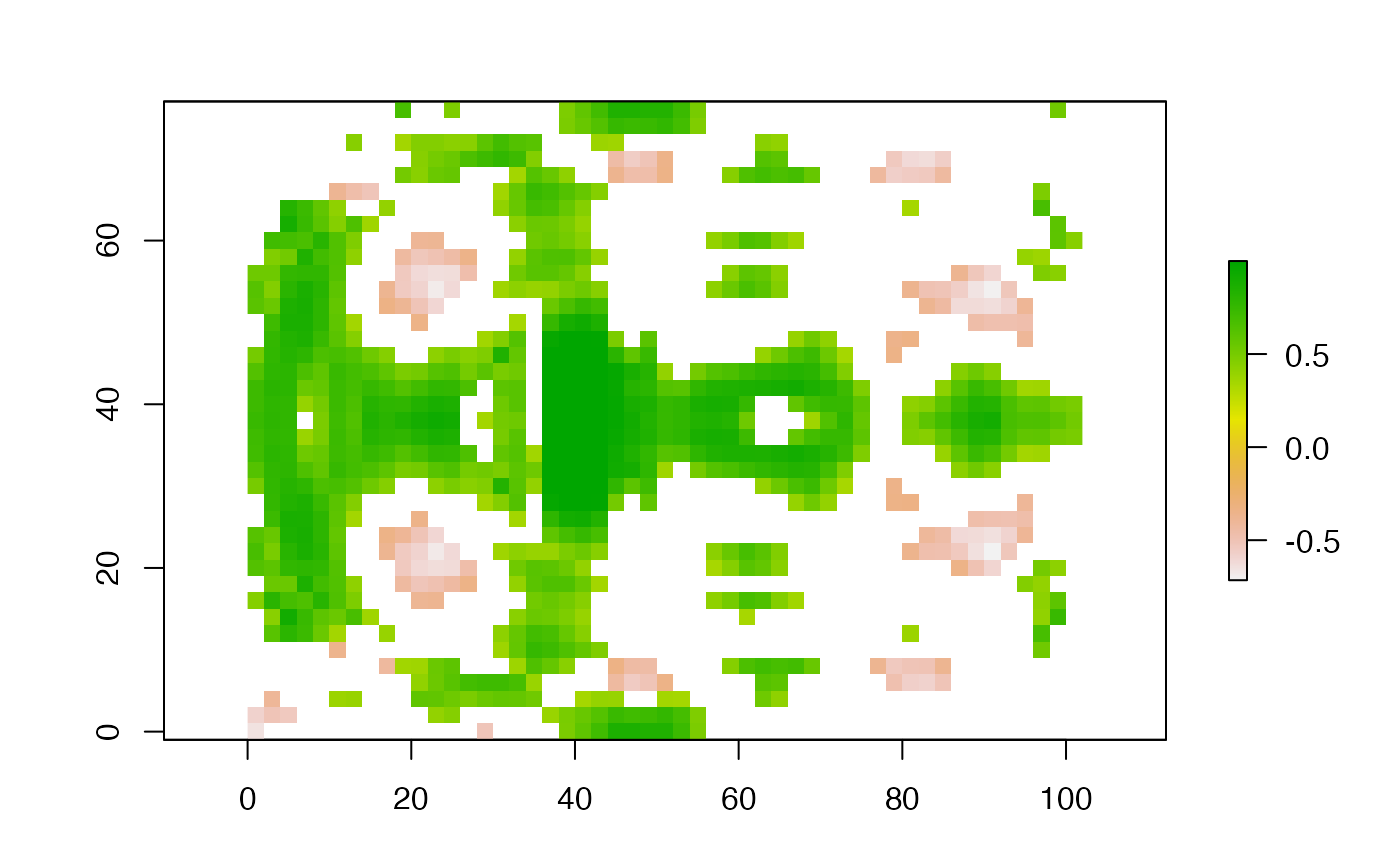Local correlation coefficient for two RasterLayer objects (using a focal neighborhood) or for two RasterStack or Brick objects (with the same number of layers (> 2))

# S4 method for RasterLayer,RasterLayer
corLocal(x, y, ngb=5,
method=c("pearson", "kendall", "spearman"), test=FALSE, filename='', ...)

# S4 method for RasterStackBrick,RasterStackBrick
corLocal(x, y,
method=c("pearson", "kendall", "spearman"), test=FALSE, filename='', ...)

## Arguments

x

RasterLayer or RasterStack/RasterBrick

y

object of the same class as x, and with the same number of layers

ngb

neighborhood size. Either a single integer or a vector of two integers c(nrow, ncol)

method

character indicating which correlation coefficient is to be used. One of "pearson", "kendall", or "spearman"

test

logical. If TRUE, return a p-value

filename

character. Output filename (optional)

...

additional arguments as for writeRaster

## Note

NA values are omitted

## Value

RasterLayer

cor, cor.test

## Examples

b <- stack(system.file("external/rlogo.grd", package="raster"))
b <- aggregate(b, 2, mean)

set.seed(0)
b[] <- flip(b[], 'y') + runif(ncell(b))
b[] <- b[] + runif(ncell(b))

x <- corLocal(b[], b[], test=TRUE )
# plot(x)

# only cells where the p-value < 0.1
plot(xm)# for global correlation, use the cor function
x <- as.matrix(b)
cor(x, method="spearman")
#>             red     green      blue
#> red   1.0000000 0.5090865 0.9423498
#> green 0.5090865 1.0000000 0.4608823
#> blue  0.9423498 0.4608823 1.0000000

# use sampleRegular for large datasets
x <- sampleRegular(b, 1000)
cor.test(x[,1], x[,2])
#>
#> 	Pearson's product-moment correlation
#>
#> data:  x[, 1] and x[, 2]
#> t = 12.743, df = 970, p-value < 2.2e-16
#> alternative hypothesis: true correlation is not equal to 0
#> 95 percent confidence interval:
#>  0.3235099 0.4312967
#> sample estimates:
#>       cor
#> 0.3786866
#>

# RasterStack or Brick objects
y <- corLocal(b, flip(b, 'y'))